## How to Calculate and Solve for Same Meridian – Same Sides of the Equator | Latitude and Longitude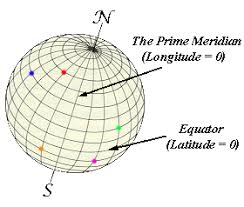The image above represents same meridian – same sides of the equator.

To compute for same meridian – same sides of the equator, two essential parameters and these parameters are value of A and value of B.

The formula for calculating same meridian – same sides of the equator:

θ = B – A

Where;

θ = Angular Difference
A = Value of A
B = Value of B

Let’s solve an example;
Find the angular difference when the value of A is 21 and the value of B is 30.

This implies that;

A = Value of A = 21
B = Value of B = 30

θ = B – A
θ = 30° – 21°
θ = 9°

Therefore, the angular difference is 9°.

Calculating the Value of B when the Angular Difference and the Value of A is Given.

B = θ + A

Where;

B = Value of B
θ = Angular Difference
A = Value of A

Let’s solve an example;
Find the value of B when the angular difference is 44 and the value of A is 5.

This implies that;

θ = Angular Difference = 44
A = Value of A = 5

B = θ + A
B = 44° + 5°
B = 49°

Therefore, the value of B is 49°.

## How to Calculate and Solve for Same Meridian – Different Sides of the Equator | Latitude and Longitude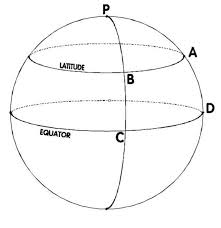The image above represents same meridian – different sides of the equator.

To compute for same meridian – different sides of the equator, two essential parameters are needed and these parameters are value of A and value of B.

The formula for calculating same meridian – different sides of the equator:

θ = A° + B°

Where;

θ = Angular Difference
A = Value of A
B = Value of B

Let’s solve an example;
Find the angular difference when the value of A is 14 and the value of B is 21.

This implies that;

A = Value of A = 14
B = Value of B = 21

θ = A° + B°
θ = 14° + 21°
θ = 35°

Therefore, the angular difference is 35°.

Calculating the Value of A when the Angular Difference and the Value of B is Given.

A = θ – B

Where;

A = Value of A
θ = Angular Difference
B = Value of B

Let’s solve an example;
Find the value of A when the value of B is 18 and the angular difference is 25.

This implies that;

θ = Angular Difference = 25
B = Value of B = 18

A = θ – B
A = 25° – 18°
A = 7°

Therefore, the value of A is 7°.

## How to Calculate and Solve for Same Latitude – Same Sides of the Prime Meridian | Latitude and Longitude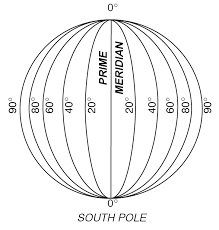The image above represents same latitude – same sides of the prime meridian.

To compute for same latitude – same sides of the prime meridian, two essential parameters are needed and these parameters are value of A and value of B.

The formula for calculating same latitude – same sides of the prime meridian:

θ = B° – A°

Where;

θ = Angular difference
B = Value of B
A = Value of A

Let’s solve an example;
Find the angular difference when the value of B is 11 and the value of A is 9.

This implies that;

B = Value of B = 11
A = Value of A = 9

θ = B° – A°
θ = 11° – 9°
θ = 2°

Therefore, the angular difference is 2°.

Calculating the Value of A when the Angular Difference and the Value of B is Given.

A = B – θ

Where;

A = Value of A
θ = Angular Difference
B = Value of B

Let’s solve an example;
Given that the value of B is 12 and the angular difference is 20. Find the value of A?

This implies that;

θ = Angular Difference = 20
B = Value of B = 12

A = B – θ
A = 12° – 20°
A = – 8°

Therefore, the value of A is – 8°.

## How to Calculate and Solve for Same Latitude – Different Sides of the Prime Meridian | Latitude and Longitude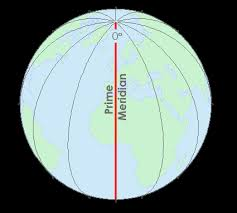The image above represents same latitude – different sides of the prime meridian.

To compute for same latitude – different sides of the prime meridian, two essential parameters are needed and these parameters are value of A and value of B.

The formula for calculating same latitude – different sides of the prime meridian:

θ = A + B

Where;

θ = angular difference
A = Value of A
B = Value of B

Let’s solve an example;
Find the angular difference when the value of A is 10 and the value of B is 12.

This implies that;

A = Value of A = 10
B = Value of B = 12

θ = A + B
θ = 10° + 12°
θ = 22°

Therefore, the angular difference is 22°.

Calculating the Value of A when the Angular Difference and the Value of B is Given.

A = θ – B

Where;

A = Value of A
θ = angular difference
B = Value of B

Let’s solve an example;
Find the value of A when the angular difference is 32 and the value of B is 14.

This implies that;

θ = angular difference = 32
B = Value of B = 14

A = θ – B
A = 32° – 14°
A = 18°

Therefore, the value of A is 18°.

## How to Calculate and Solve for the Radius of a Small Circle | Latitude and Longitude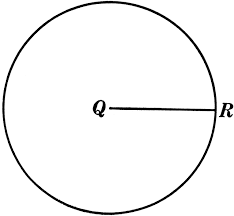The image above represents radius of a small circle.

To compute for radius of a small circle, two essential parameters are needed and these parameters are value of R and Value of the Latitude N or S of the Equator (α).

The formula for calculating radius of a small circle:

r = Rcosα

Where:

r = Radius of the small circle or Radius of Parallel of Latitude
R = Radius of the Earth
α = Value of the Latitude North or South of the Equator

Let’s solve an example;
Find the radius of a small circle when the value of R is 6400 and the value of the latitude north or south of the equator is 22.

This implies that;

R = Radius of the Earth = 6400
α = Value of the Latitude North or South of the Equator = 22

r = Rcosα
r = 6400 x cos22°
r = 6400 x 0.927
r = 5933.97

Therefore, the radius of a small circle is 5933.97 km.

Calculating the Value of the Latitude North or South of the Equator when the Radius of a Small Circle and Value of R is Given.

α = r / Rcos

Where:

α = Value of the Latitude North or South of the Equator
R = Radius of the Earth
r = Radius of the small circle or Radius of Parallel of Latitude

Let’s solve an example;
Find the value of the latitude north or south of the equator with radius of the small circle as 20 and value of R as 6400.

This implies that;

R = Radius of the Earth = 6400
r = Radius of the small circle or Radius of Parallel of Latitude =20

α = r / Rcos
α = 20 / 6400cos
α = 20 / 0.1736
α = 115.207

Therefore, the value of the latitude north or south of the equator is 115.207°.

## How to Calculate and Solve Distance Along Great Circles | Latitude and Longitude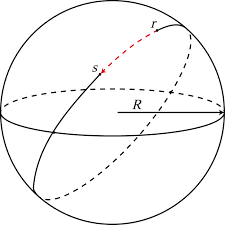The image above represents distance along great circles.

To compute for distance along great circles, two essential parameters are needed and these parameters are value of R and angular difference (θ).

The formula for calculating the distance along great circles:

y = (θ / 360) x 2πR

Where;

y = Distance Along Great Circles
θ = Angular Difference
R = Radius of the Earth

Let’s solve an example;
Find the distance along great circles when the value of R is 6400 and the angular difference is 18.

This implies that;

θ = Angular Difference = 18
R = Radius of the Earth = 6400

y = (θ / 360) x 2πR
y = (18 / 360) x (2 x π x 6400)
y = 0.05 x 40212.38
y = 2010.6

Therefore, the distance along great circles is 2010.6 km.

Calculating the Angular Difference when the Distance Along Great Circles and the Value of R is Given.

θ = y360 / 2πR

Where;

θ = Angular Difference
y = Distance Along Great Circles
R = Radius of the Earth

Let’s solve an example;
Given that the distance along great circles is 32 and the value of R is constant (6400). Find the angular difference?

This implies that;

y = Distance Along Great Circles = 32
R = Radius of the Earth = 6400

θ = y360 / 2πR
θ = (32)360 / 2π(6400)
θ = 11520 / 6.28(6400)
θ = 11520 / 40192
θ = 0.286

Therefore, the angular difference is 0.286°.

## How to Calculate and Solve for Distance Along Small circles | Latitude and Longitude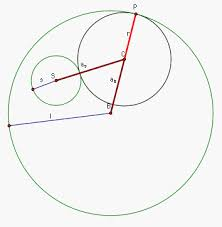The image above represents distance along small circles.

To computer for distance along small circles, two essential parameters are needed and these parameters are value of r and angular difference (θ).

The formula for calculating the distance along small circles:

x = (θ / 360) x 2πr

Where;

x = Distance Along small circles
θ = Angular Difference
r = Radius of the small circle

Let’s solve an example;
Find the distance along small circles when the angular difference is 24 and the value of r is 6.

This implies that;

θ = Angular Difference = 24
r = Radius of the small circle = 6

x = (θ / 360) x 2πr
x = (24 / 360) x 2π(6)
x = 0.066 x 37.69
x = 2.51

Therefore, the distance along small circle is 2.51 km.

Calculating the Angular Difference when the Distance Along Small Circle and the Value of r is Given.

θ = x360 / 2πr

Where;

θ = Angular Difference
x = Distance Along small circles
r = Radius of the small circle

Let’s solve an example;
Find the angular difference when the distance along small circle is 15 and the value of r is 5.

This implies that;

x = Distance Along small circles = 15
r = Radius of the small circle = 5

θ = x360 / 2πr
θ = (15)360 / 2π(5)
θ = 5400 / 31.41
θ = 171.91

Therefore, the angular difference is 171.91°.

## How to Calculate and Solve for Angle Subtended at the Centre by the Chord | Latitude and Longitude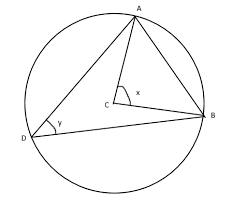The image above represents angle subtended at the centre by the chord.

To compute for angle subtended by the centre at the chord, two essential parameters are needed and these parameters are Length of the Chord of the Small Circle (l) and Radius of the Earth (R).

The formula for calculating the angle subtended by the centre by the chord:

A(2β) = 2sin-1(l2R)

Where:

A(2β) = Angle Subtended at the Centre by the Chord
R = Radius of the Earth
l = Length of the Chord of a Small Circle

Let’s solve an example;
Find the angle subtended at the centre by the chord when the radius of the earth is 6 and the length of the chord of a small circle is 4.

This implies that;

R = Radius of the Earth = 6
l = Length of the Chord of a Small Circle = 4

A(2β) = 2sin-1(l2R)
A(2β) = 2sin-1(42 x 6)
A(2β) = 2sin-1(412)
A(2β) = 2sin-1(0.33)
A(2β) = 2(19.47°)
A(2β) = 38.94

Therefore, the angle subtended at the centre by the chord is 38.94°.

## How to Calculate and Solve for Length of the Chord of a Small Circle | Latitude and Longitude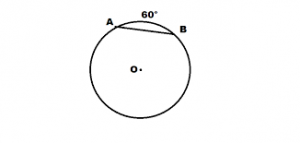The image above represents length of the chord of a small circle.

To Compute for the length of the chord of a small circle, two essential parameters are needed and these parameters are Value of r and Angular Difference (θ).

The formula for calculating the length of the chord of a small circle:

l = 2rsin(θ / 2)

Where:

l = Length of the Chord of a Small Circle
r = Radius of the small circle
θ = Angular difference

Let’s solve an example;
Find the length of the chord of a small circle when the radius of the small circle is 6 and the angular difference is 14.

This implies that;

r = Radius of the Small Circle = 6
θ = Angular difference = 14

l = 2rsin(θ / 2)
l = 2(6)sin(14 / 2)
l = (12)sin(7)
l = 12 x 0.1218
l = 1.46

Therefore, the length of the chord of the small circle is 1.46 km.

Calculating the Radius of the Small Circle when the Length of the Chord of the Small Circle and the Angular Difference is Given.

r = l / 2sin(θ / 2)

Where;

r = Radius of the small circle
l = Length of the Chord of a Small Circle
θ = Angular difference

Let’s solve an example;
Find the radius of the small circle when the length of the chord of the small circle is 35 and the angular difference is 15.

This implies that;

l = Length of the Chord of a Small Circle = 35
θ = Angular difference = 15

r = l / 2sin(θ / 2)
r = 35 / 2sin(15 / 2)
r = 35 / 2sin(7.5)
r = 35 / 2 x 0.1305
r = 35 / 0.261
r = 134.09

Therefore, the radius of the small circle is 134.09.

## How to Calculate and Solve for Shortest Distance Between Two Points | Latitude and Longitude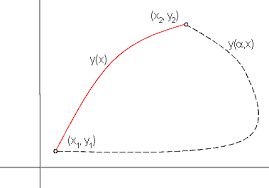The image above represents shortest distance between two points.

To compute for shortest distance between two points, two essential parameters are needed and these parameters are Value of R and Angle Subtended at the Centre by the Chord (A(2β)).

The formula for calculating the shortest distance between two points:

y = (A(2β) / 360) x 2πR

Where;

y = Shortest Distance Between Two Points
A(2β) = Angle Subtended at the Centre by the Chord
R = Radius of the Earth

Let’s solve an example;
Find the shortest distance between two points with the angle subtended at the centre by the chord as 40 and radius of the earth as 6400.

This implies that;

A(2β) = Angle Subtended at the Centre by the Chord = 40°
R = Radius of the Earth = 6400

y = (A(2β) / 360) x 2πR
y = (40 / 360) x 2π x 6400
y = 0.111 x 2π x 6400
y = 0.111 x 40212.38
y = 4468.04

Therefore, the shortest distance between two points is 4468.04 km.